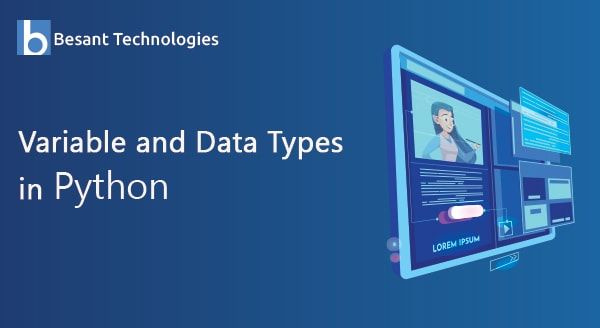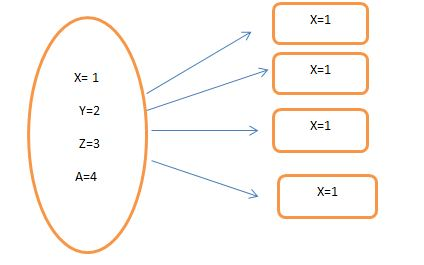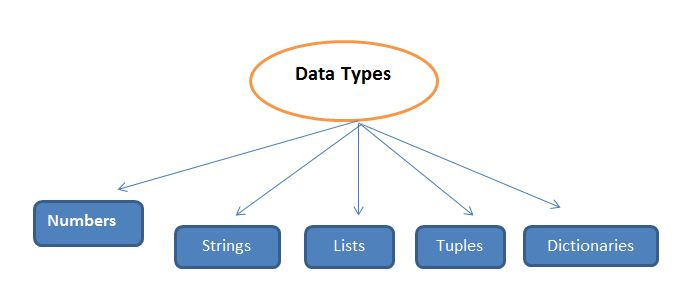Our Special Offer - Get 3 Courses at 24,999/- Only. Read more
Hire Talent (HR):+91-9707 240 250

# General# Variable and Data Types in Python

## Introduction

Python is a programming language, which is easy to understand and access. If you are a beginner, then it is important to know the fundamental things about this programming language.

In this article, we will understand the concept of variable and data type. However, before that let’s have a quick glance at the topic we are covering in this blog.

1. What Are Variables In Python?
2. Variable Definition & Declaration
3. Data Types In Python
• Numerical Data Type
• Strings
• Lists
• Tuples
• Sets
• Dictionary

## What Does Variable Mean in Python?

In python, a variable is nothing but a storage area for value. You can use the variable in the future and can also change according to your preference. According to the value you assign to your variable, the data type gets decided.

The variable gets created when you assign a value to it. There are a few rules that you need to follow when you create a variable.Let’s take look at the variable definition and declaration.

## Variable Definition & Declaration

In python, as soon as you assign value, the variable gets declared.

`X=1`

When the value 1 is assigned, a storage value for x gets allocated.

However, before that know the rules for declaring a variable, in python:

• The variable should not begin with the number. Always start with an underscore or character.
• Variable can contain alpha numeric characters.
• It is case sensitive.
• Do not use special characters.
• Do not use reserved words as a variable.

Now let’s have a look at the data types in python. In python, each value has a data type. These data types are the classes and variables that you assign are the instances (object) of these classes.

## Data Types in Python

In python, we don’t have to declare a data type. Let’s have a look at the data types that Python supports.## Number Data Type

Here, we have four subtypes of the data type. Let’s understand each of them one by one.

Int: Int stands for integer. This data type is for holding all the integer values. It can hold a value of any length unless there is space in memory. To find the class it belongs to, use type() function.

Example:

```a=11
b=22```

Float: It is for holding a decimal point value in memory. It is for storing only the number, whereas float is for floating-point values.

Example:

```a=125.12
b=150.15```

Complex: It is for holding a complex number. Complex numbers are imaginary values. For those values use ‘j’ at the end.

Example:

`x= 100+ 5j`

Boolean: Boolean is for giving an output that is either true or false. It can store either of the value.

Example:

```num= 5>4
type(num)
print (num)```

Next, let’s understand the strings.

## Strings Data Types

In python, strings are for Unicode character values. In python, there is no character data type. Here, a single character is also a string. Strings are enclosed in single quotes or double-quotes. For accessing the values of your string, use indexes, and square brackets.

Example:

```name= ‘hello’
name```

Output:

`l`

### Operations using strings

In python, you can perform a few operations and display the output accordingly.

```x= “hello”
upper() = It will display the letter is uppercase
x.lower()= It will display each character is lowercase
x.replace(‘o’, ‘O’)= It will replace the ‘o’ to ‘O’
x[1:4]= It will display strings from 1 index to 3rd index.```

After knowing about the strings, let’s cover the next data type.

## Lists

A list is an order and changeable set. It is also possible to add dummy values to your item. For declaring a list, we need to use the square brackets. In the list, you can include any data types, such as numbers, integers, or any other data type.

While understanding the data type, it is important to know the functionality and limitations that come along with it.

`myvalue= [100, 200, 300, ‘hello’]`

How to access the values from the list?

For accessing the values, we can use indexes from a string.

Example:

`myvalue[1:3]`

This will display the values of the index from 1 to 2.

### How to Add or replace values in a list?

For adding the values, use the below functionality:

`myvalue. append(‘ hello’)`

This is for adding the value at the end of the list.

`myvalue.insert(5, ‘bye’)`

This is for adding value to the 5th index.

Apart from this, there are other few operations, which you can perform.

 Method Name Functionalities clear() For removing each of the elements from the list. copy() For returning a copy of your list. remove() For removing the elements of the specified value extend() It is for adding the element to your current list. count() It can return the number of elements in the list. pop() For removing the item from the particular position. index() For accessing the index of the particular element. reverse() It can return the reserved list. sort() It is for sorts your list.

Next, sub-topic in data type, that will cover is tuples.

## Tuples

Tuples are the collection of elements is unchangeable. It is an ordered and you can access the values using the index values.  A tuple can have duplicate values. The tuple can be declared in the round brackets.

```tuple= (1, 30, 55, 60)
tuple.count(60)= The output will be 1(number of occurence).
tuple.index(30)= The output will be 1(index value, index always starts with 0).```

As said earlier, the tuple is unchangeable, so there are no many operations to perform.

Now, learn sets in python.

## Sets

A set is a collection of items, which are unordered. For declaring the set data type we can use curly brackets. It does not have any indexes. Moreover, a set cannot hold any duplicate values.

`Ourset= {1, 2, 3, 4}`

For accessing the values, you can prefer using the loop method or using the operator.

 Method Name Functionalities add() It is for adding the values in a list. update() It is adding multiple values in the list. remove() It is perfect for removing an item. clear() For clearing the items from a set. copy() It is for returning the copy of the set. difference() It can return a value with the difference of two sets. issubset() Will return if the set is a subset.

Lastly, let see the last data type in python.

## Dictionary

A dictionary is similar to the other collection of the array in python. It is an unordered and changeable set of values. It consists of a key-value pair. For declaring the dictionary, we can use a curly bracket.

dir = { ‘python’}

 Method Name Functionalities copy() It will return a copy clear() Will clear the dictionary items() Will return a set with a key value pair key() For returning a list with keys setdefault() It can return the value of specific key values() It can return a list of all values update() Updates the dictionary with all set of key-value pairs.

## Range

In python, it is a data type, which is generally helpful while using a loop. Let’s see what it is to understand it clearly.

```For a in range(100):
print(a)```

The output will be from 0-99.

Now let’s have a look at the last data type of the python.

## Type Casting

This data type is for changing one data type into another. There is an available lists of the constructor for each of the data types.

This list is

```int()

float()

list()

dict()

str()

tuple()```

These constructors are for changing one data type to another.

```x = [ 100 , 200 ,350,410]
str(x)```

This list will be changed to a string.

Using these constructors, we can manipulate the output. If I am declaring the’ x’ list into a tuple, then it will become unchangeable.

We hope you would have got a clear understanding of the variable and data types. If you are a newbie in python, then you can refer to our site, and master it in python.